Function Repository Resource:

# SylvesterMatrix

Generate the Sylvester matrix of two univariate polynomials

Contributed by: Jan Mangaldan
 ResourceFunction["SylvesterMatrix"][poly1,poly2,var] returns the Sylvester matrix of the polynomials poly1 and poly2 with respect to the variable var.

## Details

The Sylvester matrix has dimensions (m+n)×(m+n), where m and n are respectively the degrees of poly1 and poly2.
The determinant of the Sylvester matrix of two polynomials is equal to their resultant.

## Examples

### Basic Examples (1)

The Sylvester matrix of two polynomials:

 In:=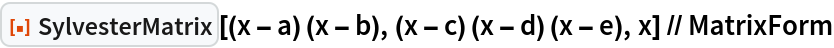Out=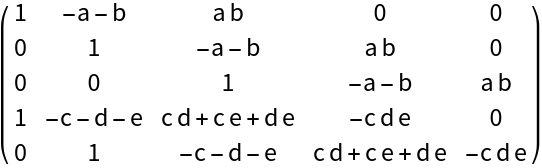### Scope (2)

The Sylvester matrix of polynomials with numeric coefficients:

 In:=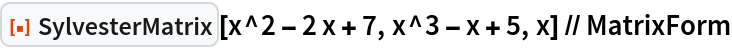Out=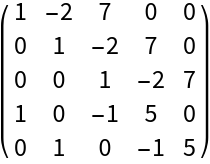The Sylvester matrix of polynomials with parametric coefficients:

 In:=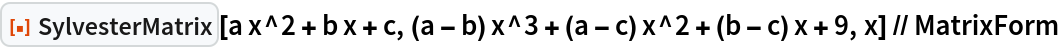Out=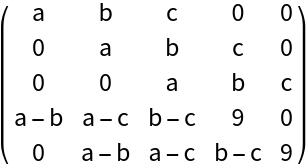### Properties and Relations (2)

The determinant of the Sylvester matrix of two polynomials is equal to their resultant:

 In:=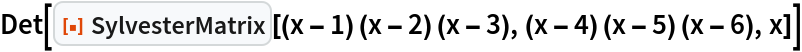Out=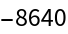In:=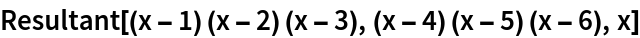Out=If one polynomial is constant, the Sylvester matrix is a diagonal matrix:

 In:=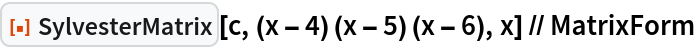Out=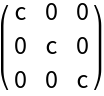## Version History

• 1.0.0 – 19 February 2021